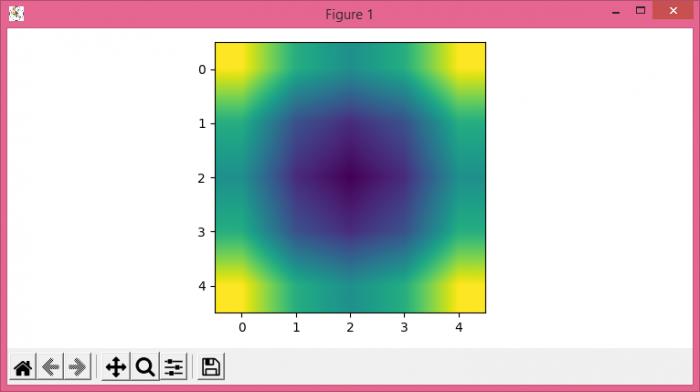# How to plot a smooth 2D color plot for z = f(x, y) in Matplotlib?

To plot a smooth 2D color plot for z = f(x, y) in Matplotlib, we can take the following steps −

• Set the figure size and adjust the padding between and around the subplots.
• Create x and y data points using numpy.
• Get z data points using f(x, y).
• Display the data as an image, i.e., on a 2D regular raster, with z data points.
• To display the figure, use show() method.

## Example

import numpy as np
from matplotlib import pyplot as plt

plt.rcParams["figure.figsize"] = [7.50, 3.50]
plt.rcParams["figure.autolayout"] = True

def f(x, y):
return np.array([i * i + j * j for j in y for i in x]).reshape(5, 5)

x = y = np.linspace(-1, 1, 5)
z = f(x, y)

plt.imshow(z, interpolation='bilinear')

plt.show()

## Output